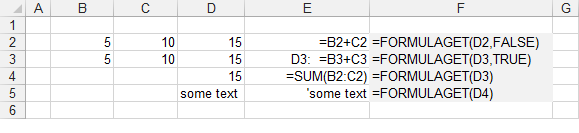### FORMULAGET

There are 2 functions.

#### FORMULAGET

Returns the formula from a particular cell.

`'rgeCell - The cell you want to check.'bCellAddressPrefix - (Optional) Whether you want the formula prefixed with the cell address.Public Function FORMULAGET(ByVal rgeCell As Range, _                    Optional ByVal bCellAddressPrefix As Boolean = False) As String       If VarType(rgeCell) = 8 And Not rgeCell.HasFormula Then       FORMULAGET = "'" & rgeCell.Formula     Else       FORMULAGET = rgeCell.Formula    End If    If rgeCell.HasArray Then       FORMULAGET = "{" & rgeCell.Formula & "}"    End If       If bCellAddressPrefix = True Then       FORMULAGET = rgeCell.Address(0, 0) & ":  " & FORMULAGET    End If End Function `

FORMULATEXT was added as a built-in function in Excel 2013.